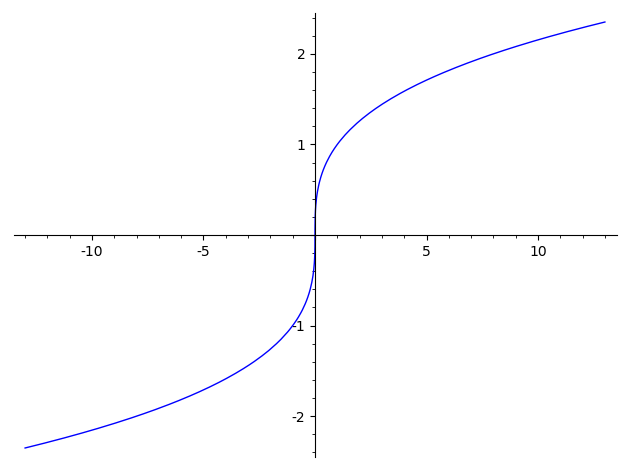# How to correctly plot x^(1/3)

sage: plot(x**(1/3))

This command produces a graph that looks like this:I was expecting a graph like this:edit retag close merge delete

1

Sort by » oldest newest most votedSageMath sometimes chooses complex cube roots, which explains this behavior.

plot(sgn(x)*abs(x)^(1/3),(x,-13,13))more

How you arrive at this function sgn(x)*abs(x)^(1/3)?

1

To get the real cube root of $-x$ (where $x>0$) you just have to add a minus sign in front of the real cube root of $x$; that's what this does.

Another way is to use the method x.nth_root(3) for real numbers x.

Using this

sage: plot(lambda x: RR(x).nth_root(3), (-1, 1))


gives the desired plot.

more

[Edited after the question was updated, clarifying it is not "why does the graph not start at zero", but "why is the negative part missing".]

Together with the unsatisfactory plot, the command

sage: plot(x^(1/3))


gives some warnings:

verbose 0 (3797: plot.py, generate_plot_points)
WARNING: When plotting, failed to evaluate function at 100 points.
verbose 0 (3797: plot.py, generate_plot_points)
Last error message: 'can't convert complex to float'


They give a clue as to what goes wrong.

By default, plot plots on the interval $[-1, 1]$ using 200 points regularly spaced along that interval to evaluate the function being plotted.

The warning that it "failed to evaluate function at 100 points", and the plot it produces, reveal that it failed to get a point to plot for all the negative values.

Here is how Sage computes powers one-third of negative numbers, depending on whether they are rational or floating-point:

sage: a = (-1)^(1/3)
sage: a
(-1)^(1/3)
sage: b = (-1.)^(1/3)
sage: b
0.500000000000000 + 0.866025403784439*I


And here is what happens when trying to convert them to floats (plot does that):

sage: float(a)
---------------------------------------------------------------------------
Traceback (most recent call last)
...
TypeError: can't convert complex to float
During handling of the above exception, another exception occurred:
TypeError: unable to simplify to float approximation
sage: float(b)
Traceback (most recent call last)
...
TypeError: unable to convert 0.500000000000000 + 0.866025403784439*I
to float; use abs() or real_part() as desired


To plot the real-cube-root function, i.e. the composition inverse of the $(x \mapsto x^3)$ bijection, there are various options.

One is indicated in @rburing's answer, and uses the sign and the absolute value to work around this limitation in Sage. It is actually part of the SageMath FAQ, see:

One other option is to plot $x = y^3$ instead of $y = x^(1/3)$.

This can be done using a parametric plot:

sage: parametric_plot((lambda t: t^3, lambda t: t), (-1, 1))This can also be done using an implicit plot since the desired graph is the locus where $x - y^3$ is zero:

sage: implicit_plot(lambda x, y: x - y^3, (-1, 1), (-1, 1))By default implicit plot comes with a frame instead of axes.

sage: implicit_plot(lambda x, y: x - y^3, (-1, 1), (-1, 1), axes=True)One can additionally remove the frame:

sage: implicit_plot(lambda x, y: x - y^3, (-1, 1), (-1, 1), axes=True, frame=False)more

what's the difference between plot and implicit_plot?

Suppose we want to visualise the set of points $(x, y)$ where $x^2 + y^2 = 1$. This cannot be put in the form $y = f(x)$. But one can think of it as the set of points where $x^2 + y^2 - 1$ is zero, and so plot it as

sage: implicit_plot(lambda x, y: x^2 + y^2 - 1, (-1, 1), (-1, 1))


It is called an implicit plot because instead of the plot of a function, it is the plot of some relation between $x$ and $y$. Some pieces of the plot could be put in the form $y = f(x)$ or $x = g(y)$, but these functions remain implicit here, as we only use some relation between $x$ and $y$ to get the plot.

So, its a function to plot relations rather than function. Thanks

Another option is to specify the xmin, xmax, ymin, ymax or just one of them:

sage: plot(x**(1/3), ymin=0)

more

Specifying these arguments still doesn't plot the correct graph.

I thought you were unhappy with the fact that the y axis was broken. Indeed, these options do not change the range of the domain of the function which is drawn. If that was the issue, then rburing's answer is the way to go.